# Multiplicative Comparison With Volume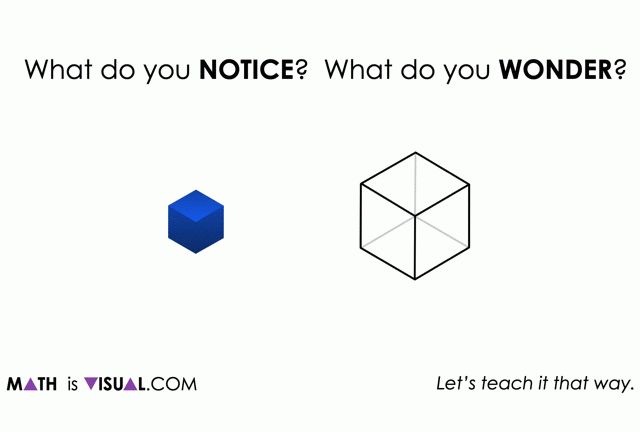Visual number talk prompts comparing the volume of 3-D figures multiplicatively using various standard and non-standard cubic units.

## In This Set of Math Visual Prompts…

Students will explore volume using non-standard cubic units and make multiplicative comparisons.

## Intentionality…

This set of visual number talk prompts is taken from the Number Talk section of Day 3 in the Make Math Moments Problem Based Unit called Can’t Get Enough Cereal. The purpose of the Number Talk is to reinforce key concepts and big ideas from this problem based math unit including:

• Volume and surface area are attributes of a three-dimensional space
• The volume of a rectangular prism is related to the edge lengths
• The volume of a rectangular prism can be determined by finding the area of the base and multiplying by the number of layers
• Rectangular prisms can be decomposed into measurable parts

Want to take a deeper dive into these concepts? Consider starting from the beginning of this 5-day problem based unit.

## Preparing to Facilitate

Present the visual math talk prompt by playing the video and prompting:

How many small cubes fit in the large cube?

Students will be asked to first estimate using their spatial reasoning followed by updating their estimates using a given multiplicative comparison between the dimensions of the small cube compared to the dimensions of the large cube.

The dimensions of the small cube and the large cube for this prompt are below:

Small Cube Dimensions: ½ unit x ½ unit x ½ unit

Large Cube Dimensions: 1 unit x 1 unit x 1 unit

Three (3) additional visual math talk prompts are shared in the Teacher Guide from Day 3 of the Can’t Get Enough Cereal problem based math unit

The following Visual Number Talk Prompt intends to Spark Curiosity and lower the floor so all students can enter into each problem.

Visual Math Talk Prompt #1

Begin playing the video to share the first visual number talk prompt and be ready to pause the video to allow for think time.

In the video, students will be prompted with:

What do you notice?

What do you wonder?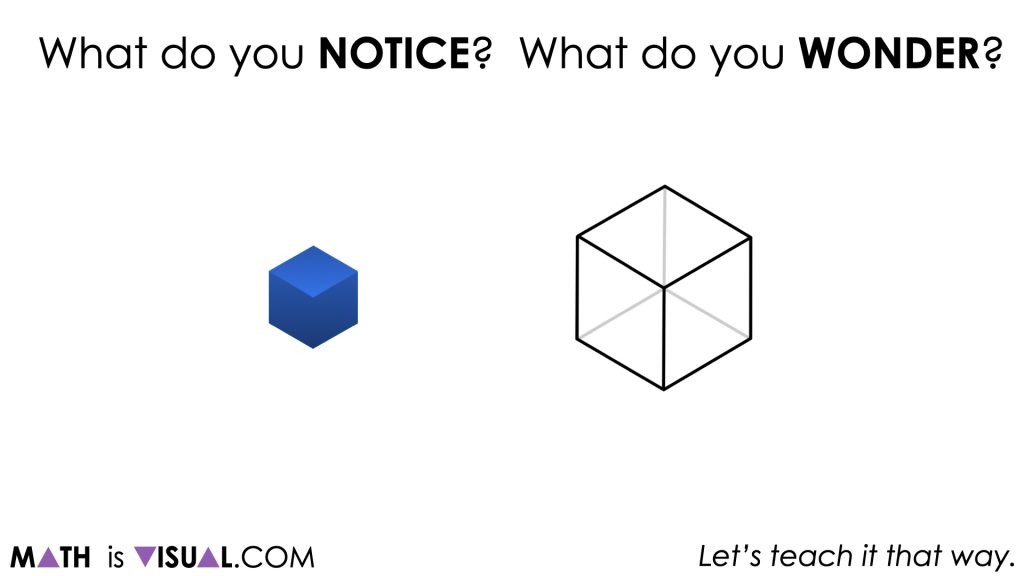Some possible observations students might share include:

• I notice that there’s a blue cube and a white cube
• I notice that the blue cube is smaller than the white cube
• I wonder how many blue cubes fit into the white cube

Of course, these are just some possible observations and many more ideas may be highlighted by your students.

Continue by asking students to consider the following:

How many blue cubes fit into a white cube?

Make an estimate.

Note that students are being asked to use visual/spatial reasoning first, as they are being asked to consider relative sizes of the cubes before being given dimensions.

Once students have begun to make estimates about the number of blue cubes that will fit into the white cube, you might first reveal the dimensions of the white cube and ask students to estimate the dimensions of the blue cube.

Students may be able to visually reason that each dimension of the white cube fits two of the blue cubes. However, some students may benefit from using wooden cubes or linking cubes to help them think through the problem. Encourage students to share their reasoning and to paraphrase the reasoning of others before revealing the next image: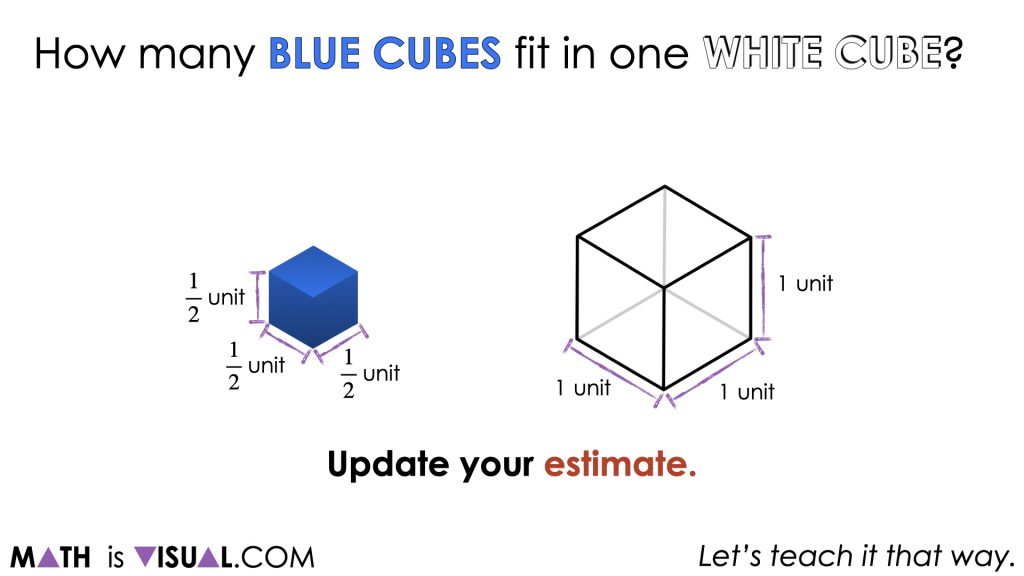As students update their estimates, be sure to ask them to reason through how they came up with their refined estimate or why they are sticking with their original estimate.

As you continue to play the visual, students will be able to see the bottom layer filling up first: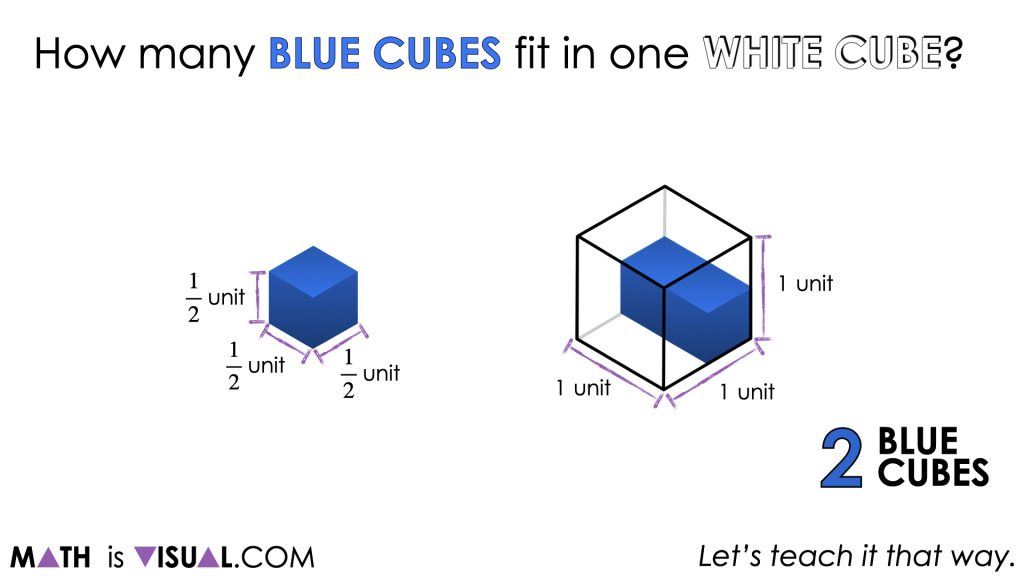Followed by the top layer to reveal that 8 blue (small) cubes can fit in the white (large) cube.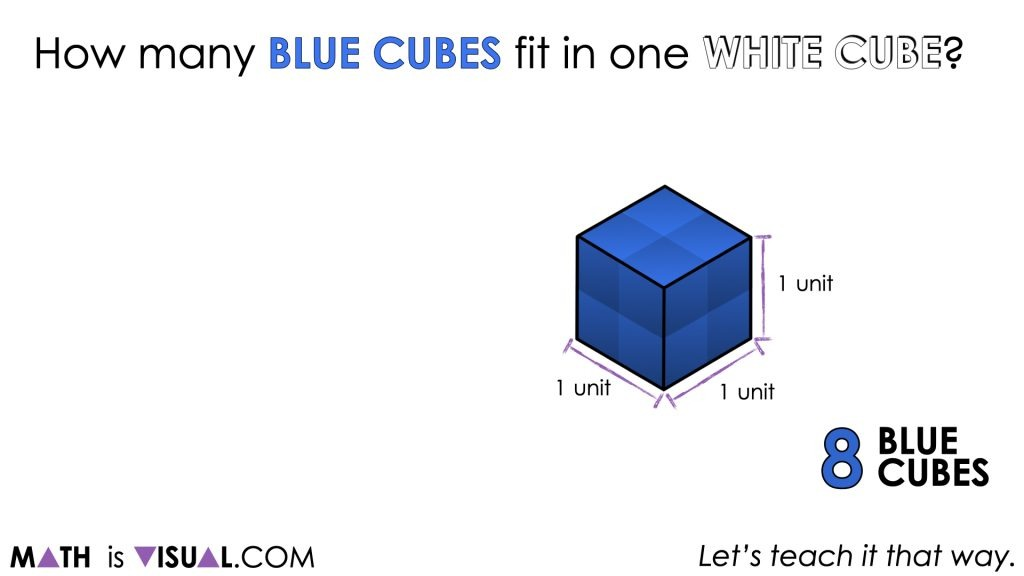What is the volume of the blue cube?

Students may see that the volume of the blue cube is ½ x ½ x ½ = ⅛  while others might initially believe that the volume must be ½ square-unit.

What connections do you see?

The goal here is for students to realize that there is a multiplicative relationship between the volume of both cubes; the larger cube is 8 times the volume of the smaller cube and the smaller cube is ⅛ the volume of the smaller cube.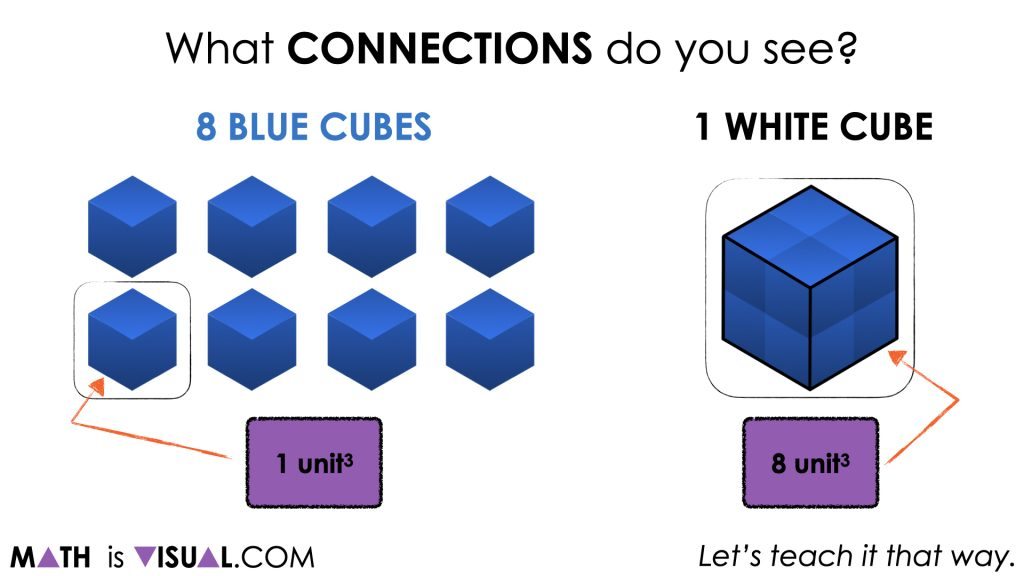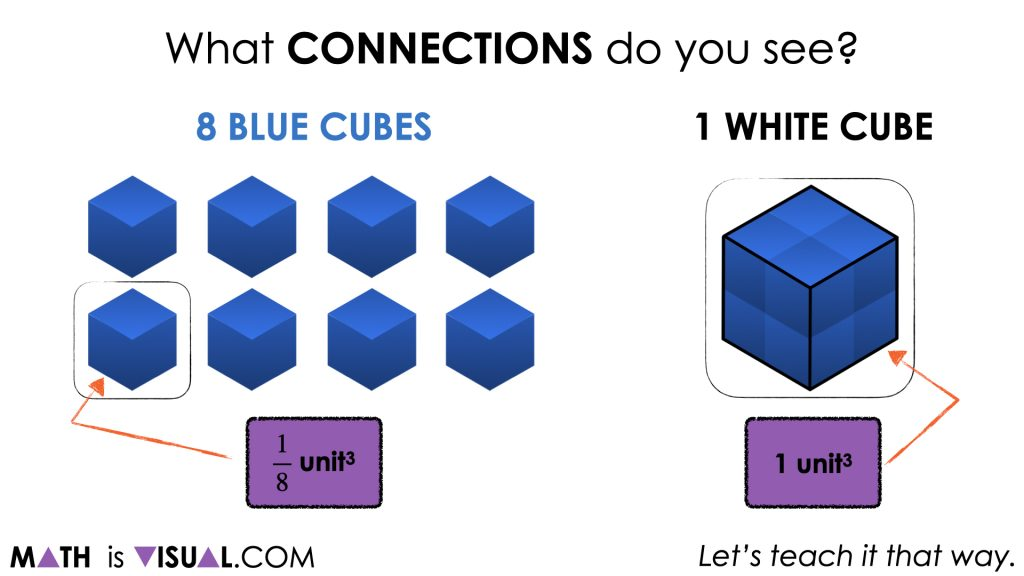## Want to Explore These Concepts & Skills Further?

Three (3) additional number talk prompts are available in Day 3 of the Can’t Get Enough Cereal problem based math unit that you can dive into now.

Why not start from the beginning of this contextual 5-day unit of real world lessons from the Make Math Moments Problem Based Units page.

Did you use this in your classroom or at home? How’d it go? Post in the comments!

Math IS Visual. Let’s teach it that way.#### kylepearce3

•Nancy Magoni says:
•kylepearce3 says: